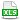HomeTechniques and TipsDeveloper Kits—BDK, EDK, RDK, RODKCombining the RDK with @RISK

# 19.12. Combining the RDK with @RISK

This article relates to discontinued products, but is retained for the benefit of our customers with existing licenses. For current information, please see Support Policy for RDK, BDK, EDK, and RODK.

Applies To:
@RISK for Excel
@RISK Developer Kit

Question:
Can the @RISK Developer Kit (RDK) be combined with interactive @RISK for Excel?

Response:
Yes. In some cases @RISK models can require hundreds of thousands of input functions and can thus take a long time to simulate. In many of these models, there's no need to represent each risk variable as a function in the spreadsheet. Rather, the necessary model values could be generated more efficiently using Visual Basic For Applications (VBA) and the Risk Developer Kit. Sampling functions in VBA, instead of placing samples and making spreadsheet calculations the traditional way using @RISK for Excel, can reduce the time and resources required.

Please download the accompanying example file. (To run this example, you will need to have the RDK installed.)

Example description:
An insurance provider sells three lines of insurance and is interested in assessing risk for the total aggregate loss across all lines. The number of claims for each line is known to follow a "Poisson" distribution. Each claim loss amount is known to follow a log-normal distribution.

Traditionally, to model this example the developer would need to have a single RiskPoisson( ) distribution to represent each line's "Total # Claims" as well as listing each RiskLognorm( ) loss distribution individually allowing the losses to be combined using a SUM( ) formula. A model of this nature could require hundreds of thousands of RiskLognorm( ) functions depending on the maximum total number of claims that are possible. Simulating a model of this size could require several minutes or even hours to process.

The @RISK Developer Kit offers an alternative approach that reduces the time required for calculating each line's total aggregate loss. For example, the developer could create a custom VBA spreadsheet function that will calculate the total aggregate loss using a FOR...NEXT loop. The distribution samples required for calculating the aggregate loss will be generated using VBA and the RDK rather than @RISK For Excel.

Use the VBA Editor (Alt+F11) to review the code in Module1 of this workbook. You will see three routines:

• A custom spreadsheet function called CalcAggregateLoss( ). This function is placed in spreadsheet cells to perform the aggregate summation without having to list each loss distribution individually; see cells C6:E6.
• An event handler for the WorkbookOpen event, which initializes the RDK.
• An event handler for the WorkbookClose event, which frees the RDK libraries.

To test this model, simply run a simulation using @RISK For Excel.

Note: This example was written before the RiskCompound( ) function was available in @RISK for Excel; see Combining Probability and Impact (Frequency and Severity).  However, the technique shown here is still valid for situations where you have a great many distributions and the simulation runs too slowly.

last edited: 2016-01-05

•Example_RDK_Claims.xls